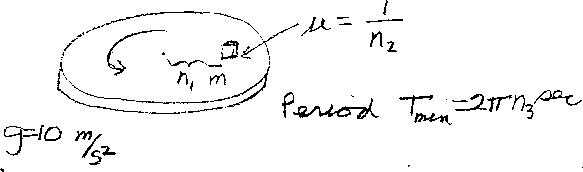Problem B13: A box is on a rotating disk, and is located a distance of n1 meters from the center. The coefficient of static friction between the box and the disk is &mu = 1/n2. What is the shortest time of rotation (Period) that the disk can have such that the box does not slide off the disk? If the shortest period T = 2 &pi n3 seconds, what is n3? For this problem, g = 10 m/s2. Note that n1 has units of meters, and n3 has units of seconds. n2 is unitless.n1 = n2 = Input n3:
If you are currently in my class, you can record your grade by entering your name and student ID number (without the leading zeros) below and clicking on "record grade".
 First Name = Last Name = ID = Problem: